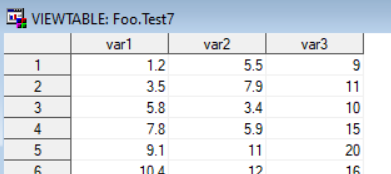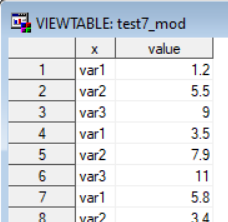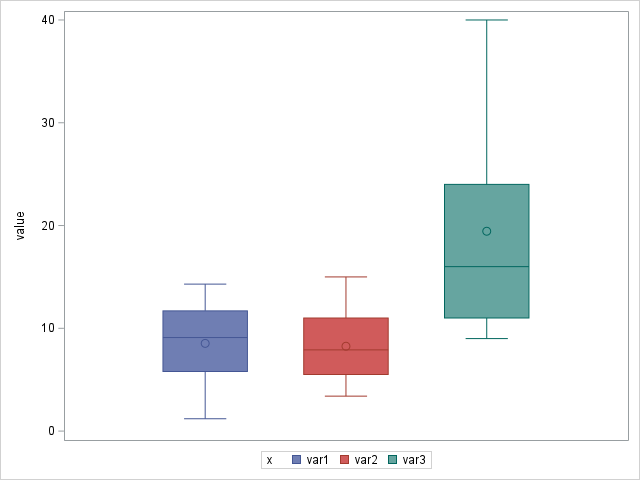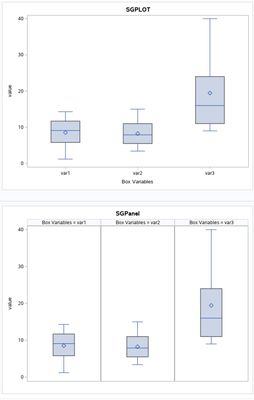## Creating boxplots

Hi all,

I will like to create a boxplot for the following 3 variables (var1, var2, var3) as in the table attached. Is there any way to achieve that? they should be displayed side by side in one diagramm

4 REPLIES 4

## Re: Creating boxplots

I will assume that you have three different columns you want in a box plot. You need to transpose this data set to a long data set so the values are in one column, and new variable contains a text string indicating the name of your original variables.

Then, use PROC SGPLOT with the VBOX statement and the CLASS= option. (For CLASS=, use the name of the new variable that contains the name of your original variables)

--
Paige Miller

## Re: Creating boxplots

Hello,

So I cannot see your data.

However, here's an example of what you are trying to do (I believe).

The Overall Statistics don't make any sense (in this specific use case) but it's just to show you something more about PROC BOXPLOT.

``````data Height;
set sashelp.class(keep=height);
run;
data Weight;
set sashelp.class(keep=weight);
run;
data HW;
set Height(rename=(height=Var_to_Plot) in=a)
Weight(rename=(weight=Var_to_Plot) in=b);
if a      then category='H';
else if b then category='W';
else;
run;

proc boxplot data=HW;
plot Var_to_Plot*category;
inset min mean max stddev /
pos    = tm;
insetgroup min max /
run;
/* end of program */``````

Cheers,

Koen

## Re: Creating boxplots

For those reluctant to download the dataset, here's what it looks like:In the simple case, you could create a box plot using code like this:

proc sgplot data=test7;
vbox var1;
run;

But the way the data is arranged in the dataset, you can't get the 3 boxplots (for var1, var2, and var3) plotted together using that simple case code.

Instead of var1, var2, and var3 being separate variables, you'll want them to be values of a single variable. Here's one way you could re-arrange the data using a simple data step:

data test7_mod (keep = x value); set test7;
x='var1'; value=var1; output;
x='var2'; value=var2; output;
x='var3'; value=var3; output;
run;

Which changes the way the data is structured to this:And then, you can create a boxplot with the following code, using the group= option (note there are multiple ways to create a box plot in SAS - this is just one of them!)

proc sgplot data=test7_mod;
vbox value / group=x;
run;## Re: Creating boxplots``````libname test  '~/test';
/* proc print data=test.test7; */
run;
data x;
set test.test7;
id=_n_;
run;
proc transpose data=x out=tran (rename=(_name_=var col1=value) drop=_label_);
by id;
var var: ;
label var='Box Variables';
run;
proc sgplot data=tran;
title 'SGPLOT';
vbox value / category=var;
/*   replace category with group for different color bars */
run;
proc sgpanel data=tran ;
title 'SGPanel';
panelby var / COLUMNS=3;
vbox value;
run;
``````
Discussion stats
• 4 replies
• 527 views
• 2 likes
• 5 in conversation